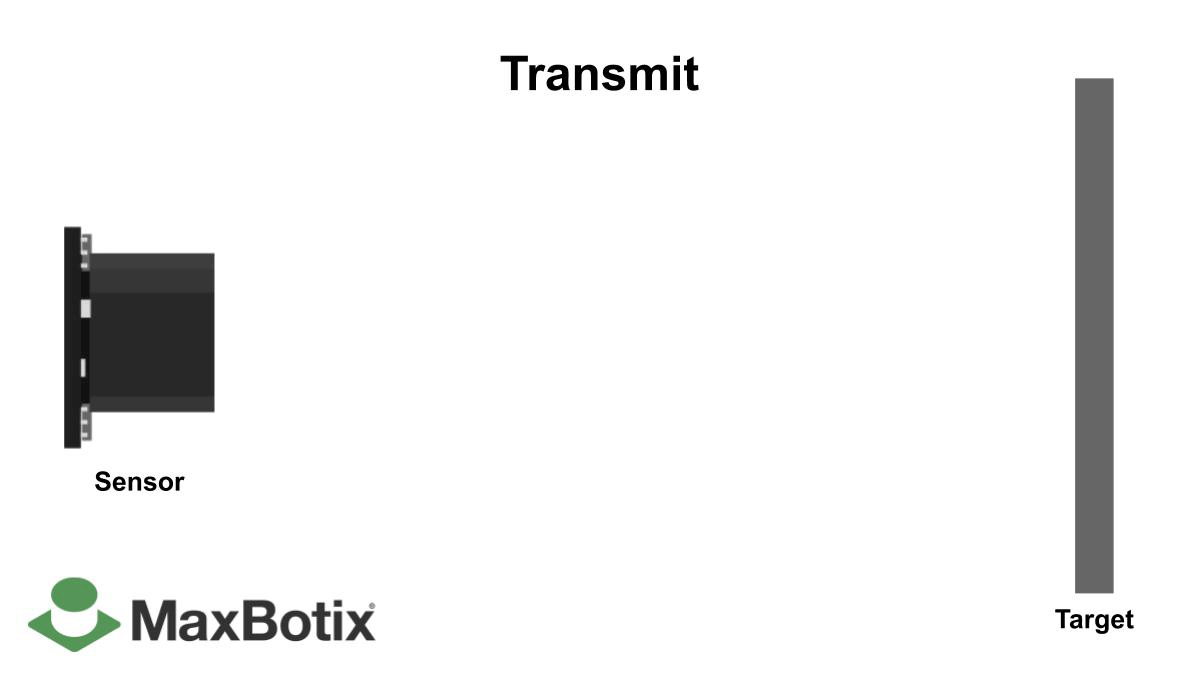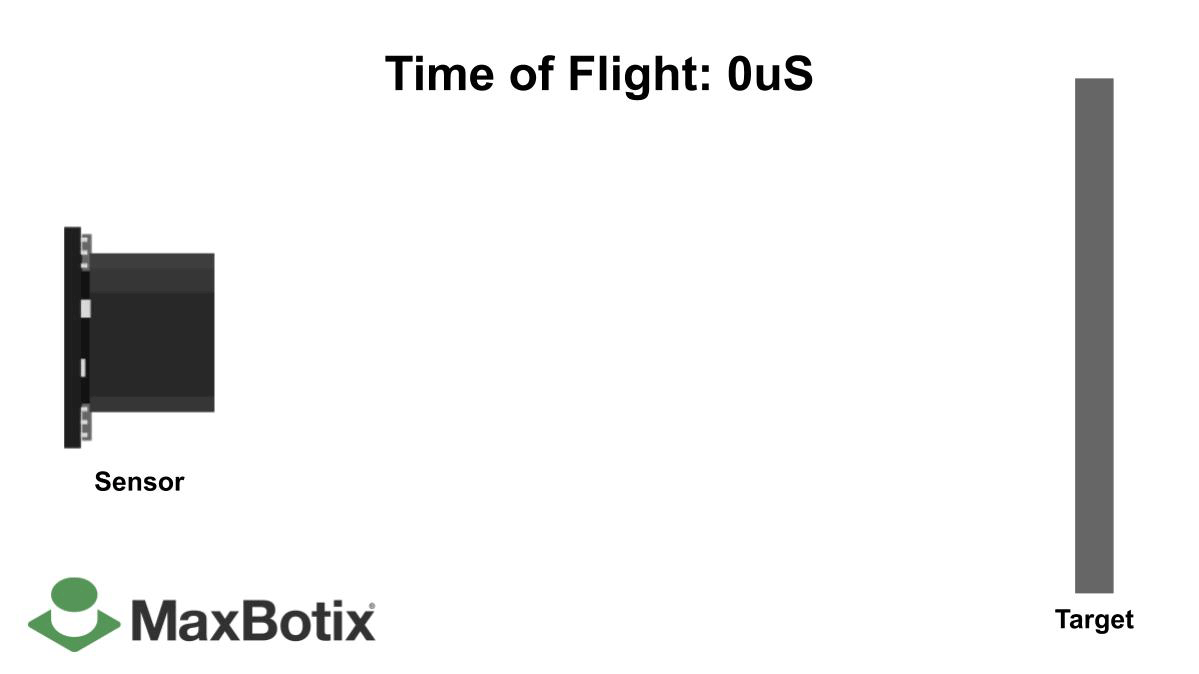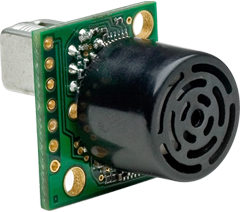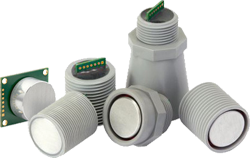# Using Pulse Width Pin 2

### Calculating Distance from Pin 2All of the MaxSonar sensors have an output waveform that is a pictorial representation of distance measured. For the majority of our sensors that waveform output is a pulse width. The Pulse Width output is 0-Vcc that shows the Time of Flight in microseconds (uS).Our standard LV-MaxSonar and XL-MaxSonar sensors assume the temperature is 22.5 degrees Celsius. These sensors do not apply temperature compensation as the air temperature changes. Our HR-MaxSonar sensors do apply automatic temperature compensation as the air temperature changes. To apply temperature compensation to your LV-MaxSonar or XL-MaxSonar part please see the Temperature Compensation PDF here.### LV-MaxSonar Sensors

To calculate the Time of Flight (ToF) pulse width for all of our LV-MaxSonar sensors use the scaling factor: 147uS/inch

The formula to convert from ToF (pulse width) is:
(ToF/147)=Ri
ToF = Time of Flight in uS (microseconds)
Ri = Range in inches

Please be aware that in order to avoid incorrect calculations ToF must be in the same unit of measurement.

If you are viewing your ToF output on an oscilloscope and have a reading of 13.23mS, you would use the formula:
(13230/147)=Ri . In this example Ri = 90

### XL-MaxSonar Sensors

To calculate the ToF pulse width for all of our XL-MaxSonar sensors use the scaling factor: 58uS/cm

The formula to convert from ToF to distance is:
(ToF/58)=Rcm
ToF = Time of Flight in uS
Rcm = Range in cm.

Please be aware that in order to avoid incorrect calculations ToF must be in the same unit of measurement.

If you are viewing your ToF output on an oscilloscope and have a reading of 10.44mS, the formula would look like:
(10440/58)=Rcm. In this example Rcm = 180### HR-MaxSonar Sensors

The scaling factor for these sensors is 1uS/mm

For this sensor family if the pulse width is 5mS, the distance to the target ranged is 5000mm.
If the pulse width to the target is 2.7mS, the distance to the target is 2700mm.

In summary for the HR-MaxSonar sensors; the represented ToF output from the sensor is equivalent to the distance of the ranged target from the sensor.

### Products Related to the Article

#### MB1220 XL-MaxSonar-EZ2The XL‑MaxSonar‑EZ2 is our most recommended indoor sensor. It offers the best balance between…

#### MB1013 HRLV-MaxSonar-EZ1The HRLV‑MaxSonar‑EZ1 is a great choice for use where sensitivity is needed along with side object…